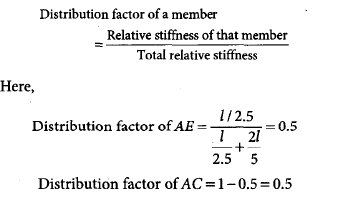Courses

# Test: Structural Analysis- 2

## 20 Questions MCQ Test GATE Civil Engineering (CE) 2022 Mock Test Series | Test: Structural Analysis- 2

Description
This mock test of Test: Structural Analysis- 2 for GATE helps you for every GATE entrance exam. This contains 20 Multiple Choice Questions for GATE Test: Structural Analysis- 2 (mcq) to study with solutions a complete question bank. The solved questions answers in this Test: Structural Analysis- 2 quiz give you a good mix of easy questions and tough questions. GATE students definitely take this Test: Structural Analysis- 2 exercise for a better result in the exam. You can find other Test: Structural Analysis- 2 extra questions, long questions & short questions for GATE on EduRev as well by searching above.
QUESTION: 1

### For a column hinged at both ends, the critical load P. Similarly, when one end of this column is made fixed and the other made free, then the critical load will become

Solution: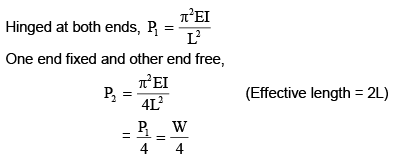QUESTION: 2

### For the structure shown below to be at equilibrium, the relation between a and b has to be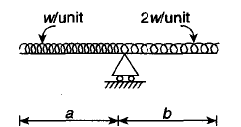Solution: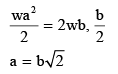QUESTION: 3

### The given figure shows a beam with its influence line for shear force and bending moment at section 1: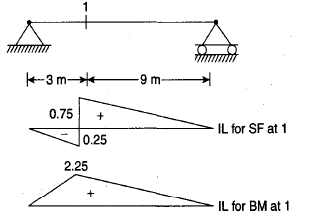The values of the shear force and bending moment at section 1 due to a concentrated load of 20 kN placed at midspan will be

Solution: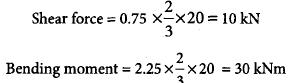QUESTION: 4

For the frame shown below; if the final moment is – 40 kNm at A and B of the column AB, then the moment MCD will be: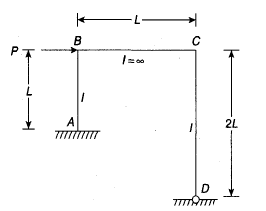Solution: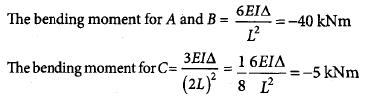QUESTION: 5

The load diagram and bending moment of a beam are shown below: The shear force at B would be:Solution: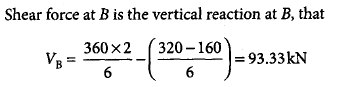QUESTION: 6

The pin-joined cantilever truss is loaded as shown below. The force is member ED is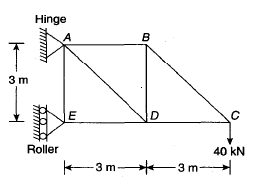Solution: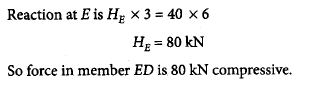QUESTION: 7

In the pin-joined plane frame shown below, the force in the member BD is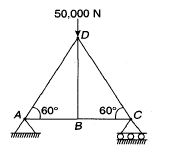Solution:

Consider the joint B
Using Equilibrium equation
Summation of vertical forces =0
Fbd=0

QUESTION: 8

The total degree of indeterminacy for the given bridge truss is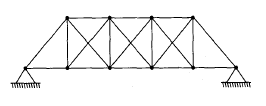Solution: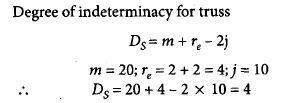QUESTION: 9

In the truss shown below, which of the following member has no force induced on it?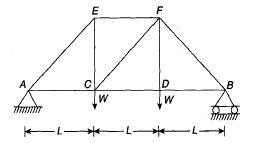Solution: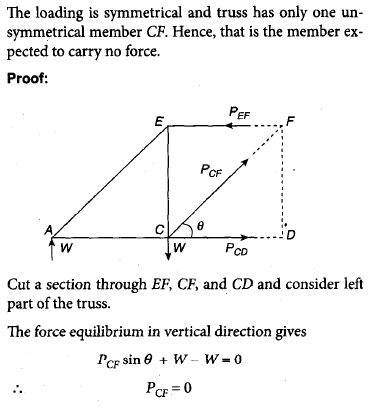QUESTION: 10

For the given frame, the distribution factors for members BC and BA at joint B are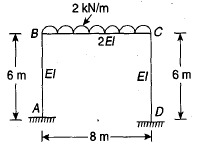Solution:
QUESTION: 11

Assertion (A): For equal distribution of moment at the support B of the beam shown in the figure, the span length x required is (3/4)L.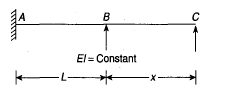Reason (R): For equal distribution of moment for the given beam, at B, I/L = 3I/4x

Solution: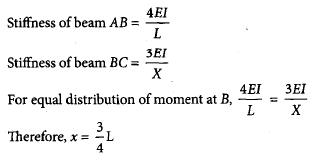QUESTION: 12

The horizontal thrust at support A of the given three hinged arch is ________ kN.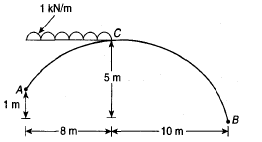Solution: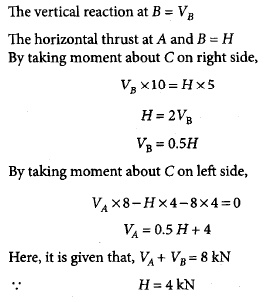QUESTION: 13

Consider a loaded trust shown in the given figure: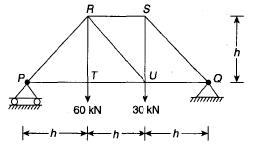Match List-I (member) with List-II (force) and select the correct answer using the codes given below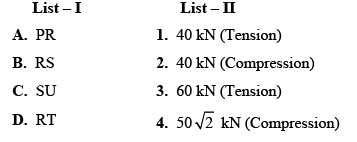Solution: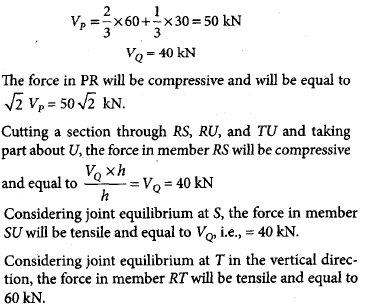QUESTION: 14

What is the ratio of the forces in the members AB, BE and AE of the pin-jointed truss shown in the figure given below: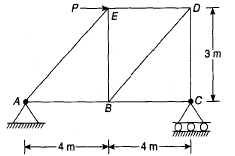Solution: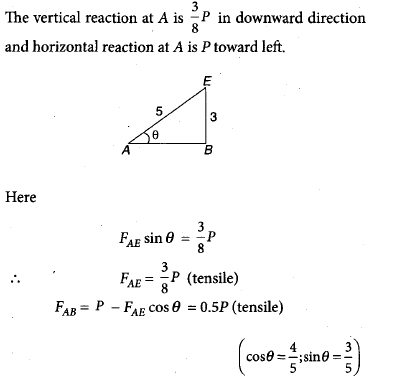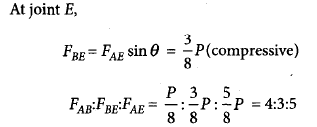QUESTION: 15

What is the degree of indeterminacy of the frame shown in the figure given below?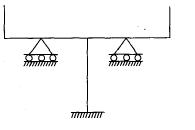Solution: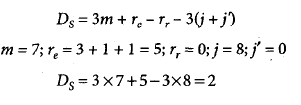QUESTION: 16

A simply supported RCC beam carrying a uniform distributed load has deflection of 100 mm at the center. When both the ends of the beam are made to be fixed, the deflection at the center will be

Solution: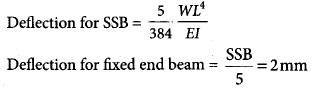QUESTION: 17

The cantilever frame, shown below, is supported by verticals at B and C and carries loads as shown in the figure. Therefore, the force in bar AE has to be __________ kg.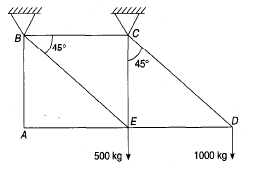Solution: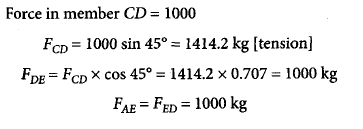QUESTION: 18

For the portal shown below, the base shear can be obtained from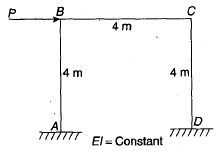Solution: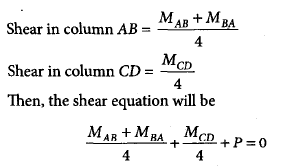QUESTION: 19

A three-hinged symmetrical arch is loaded as shown below: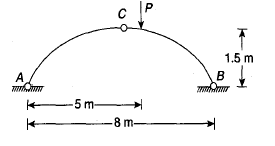Which of the following is the magnitude of the correct horizontal thrust?

Solution: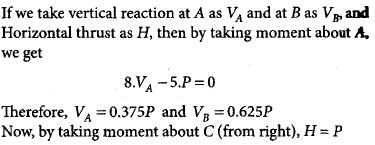QUESTION: 20

The distribution factor for members AE and AC of the given box section are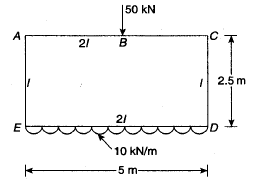Solution: